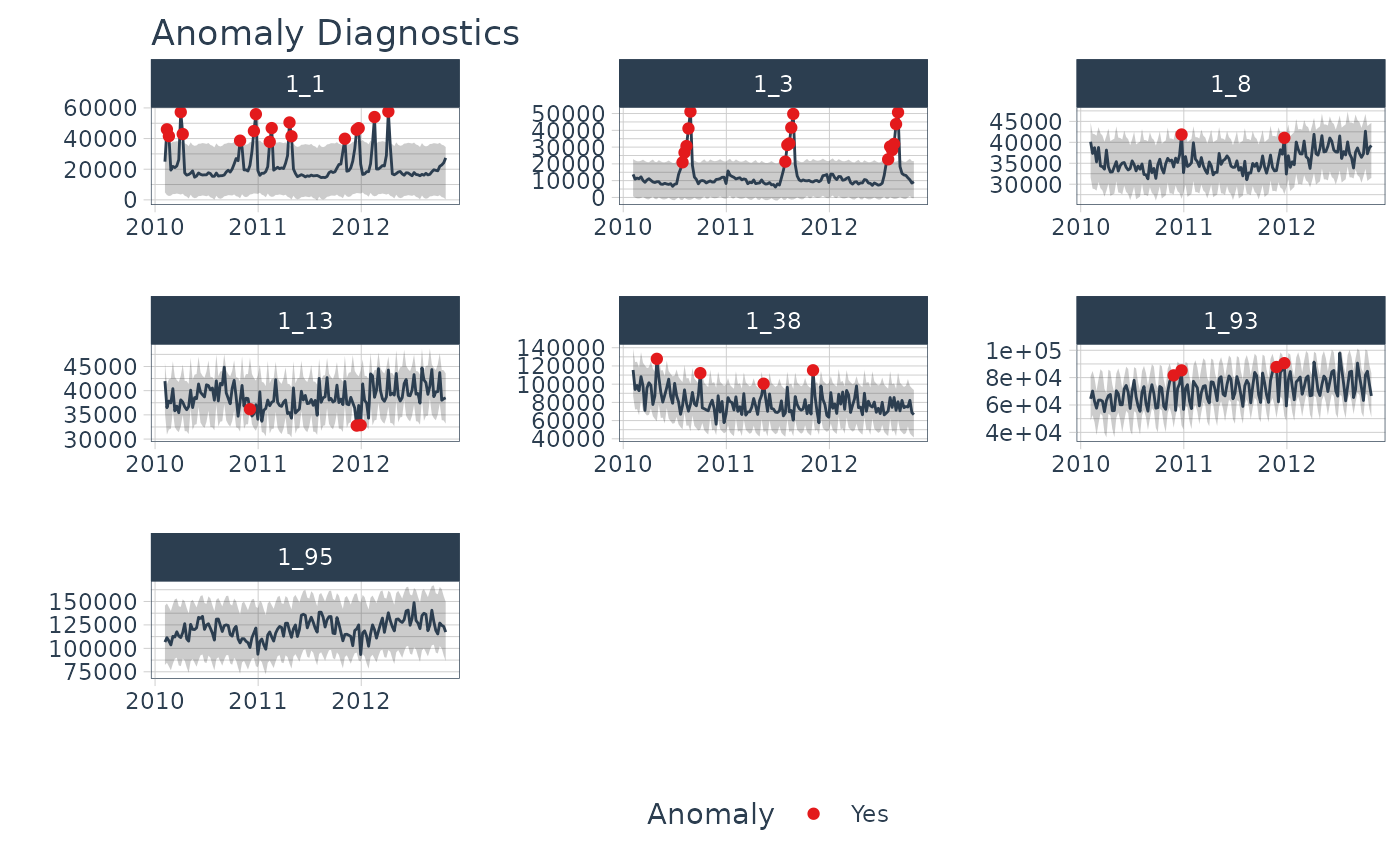An interactive and scalable function for visualizing anomalies in time series data. Plots are available in interactive plotly (default) and static ggplot2 format.

plot_anomaly_diagnostics(
.data,
.date_var,
.value,
.facet_vars = NULL,
.frequency = "auto",
.trend = "auto",
.alpha = 0.05,
.max_anomalies = 0.2,
.message = TRUE,
.facet_ncol = 1,
.facet_scales = "free",
.facet_dir = "h",
.line_color = "#2c3e50",
.line_size = 0.5,
.line_type = 1,
.line_alpha = 1,
.anom_color = "#e31a1c",
.anom_alpha = 1,
.anom_size = 1.5,
.ribbon_fill = "grey20",
.ribbon_alpha = 0.2,
.legend_show = TRUE,
.title = "Anomaly Diagnostics",
.x_lab = "",
.y_lab = "",
.color_lab = "Anomaly",
.interactive = TRUE
)

## Arguments

.data A tibble or data.frame with a time-based column A column containing either date or date-time values A column containing numeric values One or more grouping columns that broken out into ggplot2 facets. These can be selected using tidyselect() helpers (e.g contains()). Controls the seasonal adjustment (removal of seasonality). Input can be either "auto", a time-based definition (e.g. "2 weeks"), or a numeric number of observations per frequency (e.g. 10). Refer to tk_get_frequency(). Controls the trend component. For STL, trend controls the sensitivity of the LOESS smoother, which is used to remove the remainder. Refer to tk_get_trend(). Controls the width of the "normal" range. Lower values are more conservative while higher values are less prone to incorrectly classifying "normal" observations. The maximum percent of anomalies permitted to be identified. A boolean. If TRUE, will output information related to automatic frequency and trend selection (if applicable). Number of facet columns. Control facet x & y-axis ranges. Options include "fixed", "free", "free_y", "free_x" The direction of faceting ("h" for horizontal, "v" for vertical). Default is "h". Line color. Line size. Line type. Line alpha (opacity). Range: (0, 1). Color for the anomaly dots Opacity for the anomaly dots. Range: (0, 1). Size for the anomaly dots Fill color for the acceptable range Fill opacity for the acceptable range. Range: (0, 1). Toggles on/off the Legend Plot title. Plot x-axis label Plot y-axis label Plot label for the color legend If TRUE, returns a plotly interactive plot. If FALSE, returns a static ggplot2 plot.

## Value

A plotly or ggplot2 visualization

## Details

The plot_anomaly_diagnostics() is a visualtion wrapper for tk_anomaly_diagnostics() group-wise anomaly detection, implements a 2-step process to detect outliers in time series.

Step 1: Detrend & Remove Seasonality using STL Decomposition

The decomposition separates the "season" and "trend" components from the "observed" values leaving the "remainder" for anomaly detection.

The user can control two parameters: frequency and trend.

1. .frequency: Adjusts the "season" component that is removed from the "observed" values.

2. .trend: Adjusts the trend window (t.window parameter from stats::stl() that is used.

The user may supply both .frequency and .trend as time-based durations (e.g. "6 weeks") or numeric values (e.g. 180) or "auto", which predetermines the frequency and/or trend based on the scale of the time series using the tk_time_scale_template().

Step 2: Anomaly Detection

Once "trend" and "season" (seasonality) is removed, anomaly detection is performed on the "remainder". Anomalies are identified, and boundaries (recomposed_l1 and recomposed_l2) are determined.

The Anomaly Detection Method uses an inner quartile range (IQR) of +/-25 the median.

With the default alpha = 0.05, the limits are established by expanding the 25/75 baseline by an IQR Factor of 3 (3X). The IQR Factor = 0.15 / alpha (hence 3X with alpha = 0.05):

• To increase the IQR Factor controlling the limits, decrease the alpha, which makes it more difficult to be an outlier.

• Increase alpha to make it easier to be an outlier.

• The IQR outlier detection method is used in forecast::tsoutliers().

• A similar outlier detection method is used by Twitter's AnomalyDetection package.

• Both Twitter and Forecast tsoutliers methods have been implemented in Business Science's anomalize package.

1. CLEVELAND, R. B., CLEVELAND, W. S., MCRAE, J. E., AND TERPENNING, I. STL: A Seasonal-Trend Decomposition Procedure Based on Loess. Journal of Official Statistics, Vol. 6, No. 1 (1990), pp. 3-73.

2. Owen S. Vallis, Jordan Hochenbaum and Arun Kejariwal (2014). A Novel Technique for Long-Term Anomaly Detection in the Cloud. Twitter Inc.

• tk_anomaly_diagnostics(): Group-wise anomaly detection

## Examples

library(tidyverse)
library(timetk)

walmart_sales_weekly %>%
group_by(id) %>%
plot_anomaly_diagnostics(Date, Weekly_Sales,
.message = FALSE,
.facet_ncol = 3,
.ribbon_alpha = 0.25,
.interactive = FALSE)